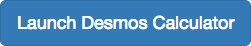## Add, Subtract, and Multiply Complex Numbers

### Learning Outcomes

• Add and subtract complex numbers
• Multiply complex numbersJust as with real numbers, we can perform arithmetic operations on complex numbers. To add or subtract complex numbers, we combine the real parts and combine the imaginary parts.

### A General Note: Addition and Subtraction of Complex Numbers

$\left(a+bi\right)+\left(c+di\right)=\left(a+c\right)+\left(b+d\right)i$

Subtracting complex numbers:

$\left(a+bi\right)-\left(c+di\right)=\left(a-c\right)+\left(b-d\right)i$

### How To: Given two complex numbers, find the sum or difference.

1. Identify the real and imaginary parts of each number.
2. Add or subtract the real parts.
3. Add or subtract the imaginary parts.

Add $3 - 4i$ and $2+5i$.

### Try It

Subtract $2+5i$ from $3 - 4i$.

## Multiplying Complex Numbers

Multiplying complex numbers is much like multiplying binomials. The major difference is that we work with the real and imaginary parts separately.

## Multiplying a Complex Number by a Real Number

Let’s begin by multiplying a complex number by a real number. We distribute the real number just as we would with a binomial. So, for example,

\begin{align}3(6+2i)&=(3\cdot6)+(3\cdot2i)&&\text{Distribute.}\\&=18+6i&&\text{Simplify.}\end{align}

### How To: Given a complex number and a real number, multiply to find the product.

1. Use the distributive property.
2. Simplify.

### Example: Multiplying a Complex Number by a Real Number

Find the product $4\left(2+5i\right)$.

### Try It

Find the product $-4\left(2+6i\right)$.

## Multiplying Complex Numbers Together

Now, let’s multiply two complex numbers. We can use either the distributive property or the FOIL method. Recall that FOIL is an acronym for multiplying First, Outer, Inner, and Last terms together. Using either the distributive property or the FOIL method, we get

$\left(a+bi\right)\left(c+di\right)=ac+adi+bci+bd{i}^{2}$

Because ${i}^{2}=-1$, we have

$\left(a+bi\right)\left(c+di\right)=ac+adi+bci-bd$

To simplify, we combine the real parts, and we combine the imaginary parts.

$\left(a+bi\right)\left(c+di\right)=\left(ac-bd\right)+\left(ad+bc\right)i$

### How To: Given two complex numbers, multiply to find the product.

1. Use the distributive property or the FOIL method.
2. Simplify.

### Example: Multiplying a Complex Number by a Complex Number

Multiply $\left(4+3i\right)\left(2 - 5i\right)$.

### Try It

Multiply $\left(3 - 4i\right)\left(2+3i\right)$.

## Contribute!

Did you have an idea for improving this content? We’d love your input.